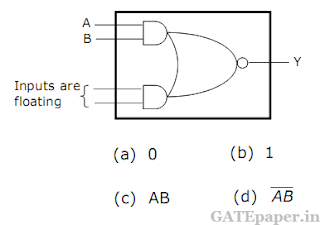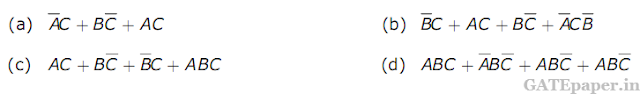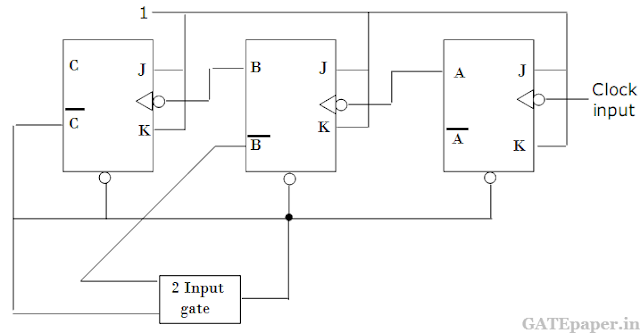### GATE 2004 ECE Video Solution on Digital Circuits (Digital Electronics)

1. A Master – Slave flip flop has the characteristic that
a. Change in the input immediately reflected in the output.
b. Change in the output occurs when the state of the master is affected
c. Change in the output occurs when the state of the slave is affected
d. Both the master and the slave states are affected at the same time.

2. The range of signed decimal numbers that can be represented by 6 bit 1’s complement form is
a. -31 to +31
b. -63 to +64
c. -64 to +63
d. -32 to +31

3. A digital system is required to amplify a binary encoded audio signal. The user should be able to control the gain of the amplifier from a minimum to a maximum in 100 increments. The minimum number of bits required to encode in straight binary is
a. 8
b. 6
c. 5
d. 7

4. Choose the correct one from among the alternatives A,B,C,D after matching an item from Group 1 with the most appropriate item in Group 2.
 Group 1 Group 2 P: Shift Register 1: Frequency division Q: Counter 2: Addressing in memory chips R: Decoder 3: Serial to Parallel data conversion

a. P – 3, Q – 2, R – 1
b. P – 3, Q – 1, R – 2
c. P – 2, Q – 1, R – 3
d. P – 1, Q – 2, R – 2

5. Figure given below shows the internal schematic of a TTL AND-OR-Invert (AOI) gate. For the inputs shown in the given figure, the output Y is6. The minimum number of 2 to 1 multiplexers required to realize a 4 to 1 multiplexer is
a. 1
b. 2
c. 3
d. 4

7. The Boolean expression AC + BC’ is equivalent to8. 11001, 1001 and 111001 correspond to the 2’s complement representation of which one of the following sets of number?
a. 25, 9 and 57 respectively
b. -6, -6 and -6 respectively
c. -7, -7 and -7 respectively
d. -25, -9 and -57 respectively

9. The 8255 programmable peripheral interface is used as described below.
(i) An A/D converter is interfaced to a microprocessor through an 8255. The conversion is initiated by a signal from the 8255 on port C. A signal on port C. A signal on port C causes data to be strobed into port A.
(ii) Two computers exchange data using a pair of 8255s. Port A works as a bidirectional data port supported by appropriate handshaking signals.
The appropriate modes of operation of the 8255 for (i) and (ii) would be
a. Mode 0 for (i) and Mode 1 for (ii)
b. Mode 1 for (i) and Mode 0 for (ii)
c. Mode 2 for (i) and Mode 0 for (ii)
d. Mode 2 for (i) and Mode 1 for (ii)
Solution :

10. The number of memory cycles required to execute the following 8085 instructions
(i) LDA 3000H
(ii) LXI D, F0F1H
Would be
a. 2 for (i) and 2 for (ii)
b. 4 for (i) and 3 for (ii)
c. 3 for (i) and 3 for (ii)
d. 3 for (i) and 4 for (ii)
Solution :

11. In the modulo – 6 ripple counter shown in the given figure, the output of the 2 input gate is used to clear the JK flip flops.The 2 input gate is
a. NAND gate
b. NOR gate
c. OR gate
d. AND gate

12. Consider the sequence of 8085 instructions given below.
LXI H, 9258H
MOV A, M
CMA
MOV M, A
Which one of the following is performed by this sequence of instructions?
a. Contents of location 9258 are moved to the accumulator
b. Contents of location 9258 are compared with the contents of the accumulator
c. Contents of location 9258 are complemented and stored in location 9258
d. Contents of location 5892 are complemented and stored in location 5892.

13. A Boolean function f of two variables x and y is defined as follows:
f(0,0) = f(0,1) = f(1,0) = 1; and f(1,0) = 0
Assuming complements of x and y are not available, a minimum cost solution for realizing f using only 2 input NOR gates and 2 input OR gates (each having unit cost) would have a total cost of
a. 1 unit
b. 4 units
c. 3 unit
d. 2 units

14. It is desired to multiply the numbers 0AH by 0BH and store the result in the accumulator. The numbers are available in registers B and C respectively. A part of the 8085 program for this purpose is given below:
MVI A, 00H
LOOP: ..............
..............
..............
HLT
END
The sequence of instructions to complete the program would be
a. JNZ LOOP, ADD B, DCR C
b. ADDB, JNZ LOOP, DCR C
c. DCR C, JNZ LOOP, ADD B
d. ADD B, DCR C, JNZ LOOP